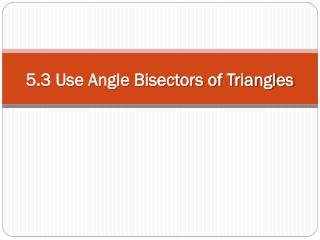DownloadDownload Presentation5.3 Use Angle Bisectors of Triangles

# 5.3 Use Angle Bisectors of Triangles

Download Presentation## 5.3 Use Angle Bisectors of Triangles

- - - - - - - - - - - - - - - - - - - - - - - - - - - E N D - - - - - - - - - - - - - - - - - - - - - - - - - - -
##### Presentation Transcript

1. 5.3 Use Angle Bisectors of Triangles

2. Objectives • Use properties of angle bisectors • Locate the incenter

3. Vocabulary Recall, an angle bisector is a ray that divides an angle into two congruent adjacent angles. The distance from a point to a line is the length of the perpendicular segment from the point to the line. b

4. Angle Bisector Theorems • Theorem 5.5 - Angle Bisector Theorem: If a point is on the bisector of an angle, then it is equidistant from the two sides of the angle. Since AD is an  bisector, then DE ≅ DF. • Theorem 5.6 – Converse of the Angle Bisector Theorem: If a point is in the interior of an angle and is equidistant from the sides of the angle, then it lies on the bisector of the angle. E F

5. SOLUTION Because JG  FGand JH FHand JG =JH = 7, FJ bisects GFH by the Converse of the Angle Bisector Theorem. So, mGFJ =mHFJ= 42°. Example 1 EXAMPLE 1 Use the Angle Bisector Theorems Find the measure of GFJ.

6. A soccer goalkeeper’s position relative to the ball and goalposts forms congruent angles, as shown. Will the goalie have to move farther to block a shot toward the right goalpost Ror the left goalpost L? The congruent angles tell you that the goalie is on the bisector of  LBR. By the Angle Bisector Theorem, the goalie is equidistant from BRand BL. So, the goalie must move the same distance to block either shot. Example 2 EXAMPLE 2 SOLUTION

7. For what value of xdoes Plie on the bisector of  A? CP BP = 2x –1 x + 3 = 4 = x Example 3 EXAMPLE 3 Use algebra to solve a problem SOLUTION From the Converse of the Angle Bisector Theorem, you know that if Plies on the bisector of Athen Pis equidistant from the sides of A, so BP =CP. Set segment lengths equal. Substitute expressions for segment lengths. Solve for x. Point Plies on the bisector of  Awhen x = 4.

8. B P A C ANSWER ANSWER ANSWER 11 5 15 More Practice Problems GUIDED PRACTICE For numbers1–3, find the value of x. 1. 2. B P A C P 3. B C A

9. Do you have enough information to conclude that QSbisects  PQR? Explain. ANSWER No; you need to establish thatSR QRand SP  QP. Example 4 GUIDED PRACTICE

10. Vocabulary and Another Theorem • Theorem 5.7 Concurrency of Angle Bisectors of a Triangle: The angle bisectors of a triangle intersect at a point that is equidistant from the sides of the triangle. • The point of concurrency of the three angle bisectors of a triangle is called the incenter of the triangle. • The incenter always lies inside the triangle.

11. Example 5 EXAMPLE 4 Use the concurrency of angle bisectors In the diagram, Nis the incenterof ABC. Find ND. SOLUTION By the Concurrency of Angle Bisectors of a Triangle Theorem, the incenterNis equidistant from the sides of  ABC. So, to find ND, you can find NFin  AF. Use the Pythagorean Theorem, a2 + b2 = c2.

12. 2 2 2 c = a + b 400 = 2 NF + 256 2 144 = NF 12 = NF 2 2 2 20 = NF + 16 Example 5 (continued): EXAMPLE 4 Pythagorean Theorem Substitute known values. Multiply. Subtract 256 from each side. Take the positive square root of each side. Because NF = ND, ND = 12.